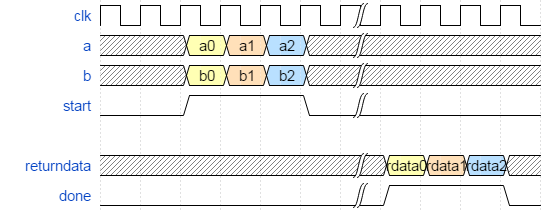ID 683456
Date 12/19/2022
Public

## 6.3.2. Comparison of Explicit and Enqueued Function Calls

The ihc_hls_enqueue and ihc_hls_enqueue_noret functions allow a new invocation of a component to start every cycle if the component can be pipelined with a component initiation interval (II) of one. If the component II is greater than one, then the next component invocation starts after II number of cycles.

Waveform Diagram of the Signals for Component dut Without Enqueue Function Calls illustrates the waveform of the signals for the component dut. The testbench does not include any enqueue function calls.

#include "HLS/hls.h"
#include <stdio.h>

component int dut(int a, int b) {
return a*b;
}

int main (void) {

int x1, x2, x3;
x1 = dut(1, 2);
x2 = dut(3, 4);
x3 = dut(5, 6);

printf("x1 = %d, x2 = %d, x3 = %d\n", x1, x2, x3);

return 0;
}
Figure 2. Waveform Diagram of the Signals for Component dut Without Enqueue Function CallsWaveform Diagram of the Signals for Component dut With Enqueue Function Calls illustrates the waveform of the signals for the component dut when the testbench includes enqueue function calls. Observe how the component is passed new data each clock cycle, and compare this waveform with the earlier waveform.
#include "HLS/hls.h"
#include <stdio.h>

component int dut(int a, int b) {
return a*b;
}

int main (void) {

int x1, x2, x3;
ihc_hls_enqueue(&x1, &dut, 1, 2);
ihc_hls_enqueue(&x2, &dut, 3, 4);
ihc_hls_enqueue(&x3, &dut, 5, 6);

ihc_hls_component_run_all(&dut);

printf("x1 = %d, x2 = %d, x3 = %d\n", x1, x2, x3);

return 0;
}
Figure 3. Waveform Diagram of the Signals for Component dut With Enqueue Function Calls